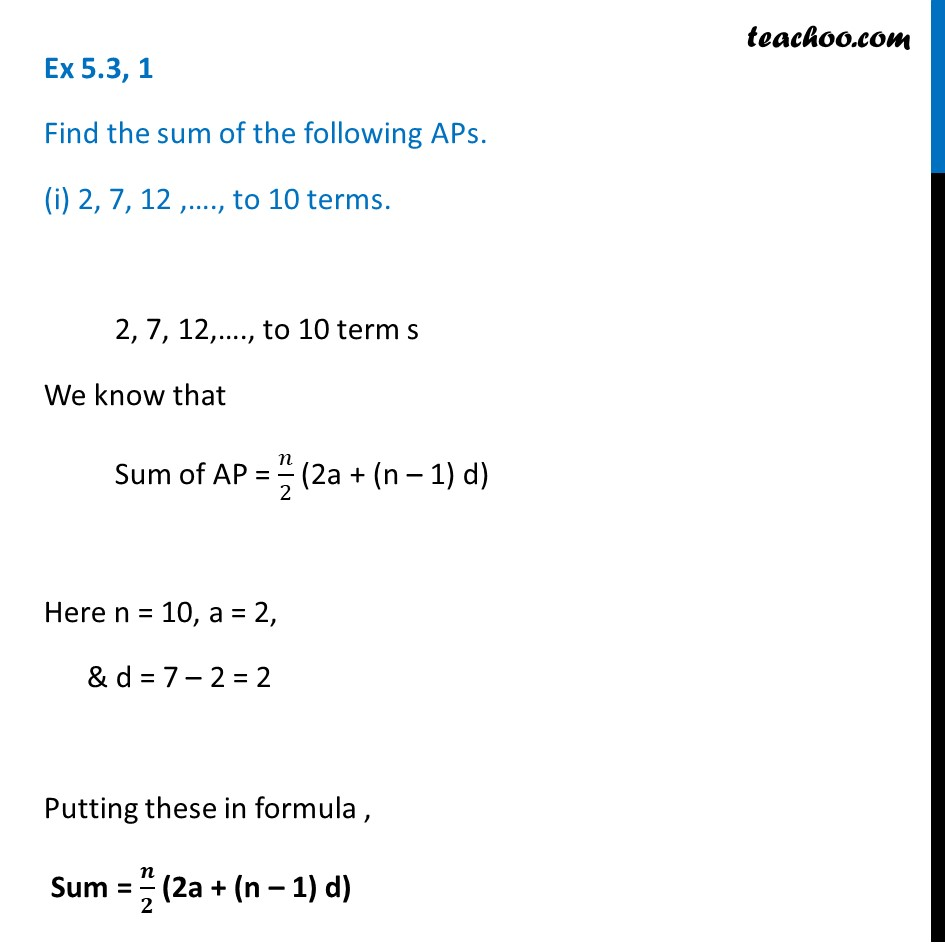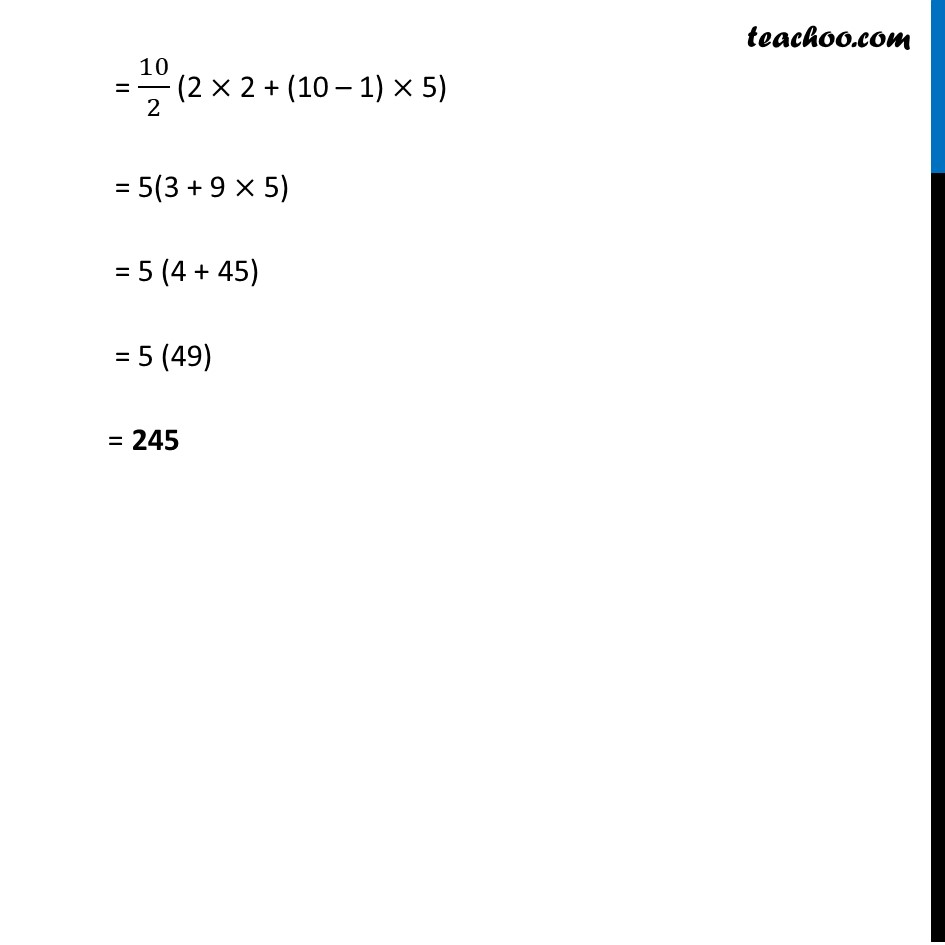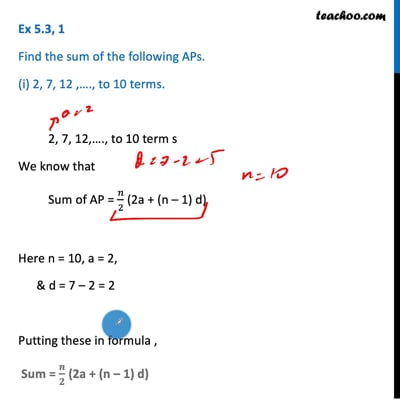Finding sum of n terms

Chapter 5 Class 10 Arithmetic Progressions (Term 2)
Concept wiseThis video is only available for Teachoo black users

### Transcript

Ex 5.3, 1 Find the sum of the following APs. (i) 2, 7, 12 ,…., to 10 terms. 2, 7, 12,…., to 10 term s We know that Sum of AP = 𝑛/2 (2a + (n – 1) d) Here n = 10, a = 2, & d = 7 – 2 = 2 Putting these in formula , Sum = 𝒏/𝟐 (2a + (n – 1) d) = 10/2 (2 × 2 + (10 – 1) × 5) = 5(3 + 9 × 5) = 5 (4 + 45) = 5 (49) = 245